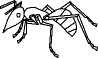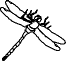EnchantedLearning.com is a user-supported site.
As a bonus, site members have access to a banner-ad-free version of the site, with print-friendly pages.

 You might also like: Divide Twelve Farm Animals Worksheet Printout Math: Insects Divide Fish by Five Worksheet Printout Divide Fifteen Icy Items Worksheet Printout Divide Ten Pets Worksheet Printout Today's featured page: Food-Related Inventors and Inventions

 Go to the AnswersMath Pages EnchantedLearning.com Divide Groups of Bugs Divide the bugs into groups to do division. Visual Division PagesDivision Pages

 Divide the ants into two equal groups.How many ants are there? How many groups of ants are there? How many ants are in each group?
10 ÷ 2 =

 Divide the moths into five equal groups.How many moths are there? How many groups of moths are there? How many moths are in each group?
10 ÷ 5 =

 Divide the dragonflies into four equal groups.How many dragonflies are there? How many groups of dragonflies are there? How many dragonflies are in each group?
12 ÷ 4 =

## Enchanted Learning Search

 Search the Enchanted Learning website for: